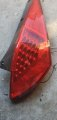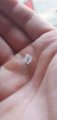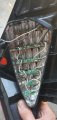# Resistor selection - Custom taillight project

#### Ea73xa

Joined Apr 7, 2019
9
Hello all.

First time poster
Long time visitor

I was wondering if someone with a Bit more notice than myself could help me with a project I'm currently undertaking.

It's a tailight of a Nissan 350z
Currently creating a prototype out of a single damaged unit with the hopes of making a fresh set. If only I could get the resistor values correct.

Iv done small led installs with resistors and such but this seems to be a Bit above me.

I have included some photos

This is the tailight.
It has 20 less that all come on at once.
Dimmer for driving lights
And then brighter for braking. Pretty standard stuff.And this is a quick mock up of what I wish to create.I wish to have these 10 Leds as driving lights

With the rest lighting up brighter when applying the brakes.

I have 2 power sources to the light itself:
The earth
One for driving
And the one for the brakes.

I'm guessing I need to wire these into 2 separate circuits.
My issue is working out what resistor to use for each so I can achieve the desired brightness for each stage of the light.

I burnt out plenty of the original Leds testing the circuits.

So I'm fitting new ones.
SuperFlux in red.And this is the guts of it.

I gutted the original wiring setup, traced the tracks and isolated each led so they can be wired in seriesCan anyone shed some light on what resistors I would need for this to work?

The feed voltage to the lights is 12.5v
And for memory the Sfled are .5w each

Thank you
And sorry for the noob question

#### Picbuster

Joined Dec 2, 2013
1,041
I assume the all in parallel. that implies;
each led has a resistor in serial.
resulting in maximum_car_voltage - Voltage_over_led = the voltage over resistor.
Voltage_over_led is type depended but state as 1.9 to 2.1 Volt @ 20mA.
Assume that your car does 14V while running
voltage over resistor = 14 - 2.1 = 11.9Volt @ 20mA
Resistor = 11.9/0,020 = 595 Ohm
power in resistor i ^2 *R ==> 0,02 ^2 x 595 = 0,238 watt
However 595 Ohm is not a common value so use 600 Ohm
The current will be a bit less.

I don't know where you are located but in some countries is modifying anything including car paint colour is not allowed.
Any change is violating the governments road acceptance for that motor.

Picbuster

#### Ea73xa

Joined Apr 7, 2019
9

This 600 ohm resistor is that one resistor for each Led or is that one 600ohm for every strip of 5?

Forgive me but the maths of this is bewildering

Would that also be the dimmer running light circuit?
Because I Am going to assume the other remaining Leds that I'll use for braking will require being brighter than the other ones used for driving

And I'm from Australia
Our laws differ from state to state
But this isn't really considered an illegal modification

#### Picbuster

Joined Dec 2, 2013
1,041

This 600 ohm resistor is that one resistor for each Led or is that one 600ohm for every strip of 5?

Forgive me but the maths of this is bewildering

Would that also be the dimmer running light circuit?
Because I Am going to assume the other remaining Leds that I'll use for braking will require being brighter than the other ones used for driving

And I'm from Australia
Our laws differ from state to state
But this isn't really considered an illegal modification
Yes for each led and max intensity.
If you use a string (serial) then change the formulae from Voltage_over_led to n * Voltage_over_led. ( n number of leds in a string)
and recalculate.
Result is a lower resistor value.

If you want to reduce change the resistor value to a higher one.
Remember: there is no linear relation between current and light intensity. ( look at the data sheet).

Picbuster
PS: electronics is math. Without calculations no reliable working circuits.

•Ea73xa

#### Ya’akov

Joined Jan 27, 2019
7,023
Hello, @Ea73xa.

It’s very hard to tell from the photo precisely how the LEDs are wired.

I suspect there are serial circuits of some number that are then wired in parallel, but it’s nearly impossible for me to follow the wiring.

To help you keep from burning more LEDs, it would be extremely useful if you could trace the circuit and draw a schematic. It makes a big difference how they are wired, and it’s definitely worth your time to learn to trace the circuit.

#### Ea73xa

Joined Apr 7, 2019
9
Picbuster

I failed maths unfortunately
Just something my brain cannot wrap around
I know it in basic forms
But completely understand that it's a requirement for this type of thing
That's why I Am seeking assistance.

Yaakov

Best I can muster at work for now
I plan to use 2 separate circuits.
One for brake
The other for driving

The driving requires dimmer light than braking

Hope my scribble can help#### Picbuster

Joined Dec 2, 2013
1,041
10 in serial will have a Vf of 10 x 2.1 = 21 V approx your car battery is normally lower (13.8V with running engine).

leds will not work as expected.

Picbuster

#### Ea73xa

Joined Apr 7, 2019
9
Guess it's a resistor with each led then

#### djsfantasi

Joined Apr 11, 2010
8,783
A resistor for every LED is optimal.

But you could wire them in a series/parallel circuit. Wire two groups of 5. The voltage over LEDs would be 5 x 2.1 or 10.5 volts. With a running voltage of 13.8, your resistor would have to drop 3.3 volts. 3.3 volts divided by 20mA is 165 ohms. The closest resistor is 180 ohms.

So, wire two groups of 5 with a 180 ohm resistor in series. Then connect each group in parallel.

•Ea73xa

#### Ea73xa

Joined Apr 7, 2019
9
Djsfantasi

Now that's an answer I can understand!
Thank you

I'm also going to hazard a guess and say this if for maximum light output?

#### djsfantasi

Joined Apr 11, 2010
8,783
Djsfantasi

Now that's an answer I can understand!
Thank you

I'm also going to hazard a guess and say this if for maximum light output?
Yes, but my calculations were based on the data given in other posts. You may want to double check these values.
• The Vf of the LEDs is 2.1V
• The current of the LEDs is 20mA. You may want to recalculate with a lower current, say 15mA
• The voltage of the vehicle is 13.8V

Using my or PicBuster’s posts, you should be able to perform them yourself. I’d particularly substitute 15mA and recalculate. You never want to run LEDs at their maximum current. They don’t like it and you may find that they will fail on you. Post your attempts here, and someone will double check your work.

Do you have a benchtop power supply? I recommend testing each string on the bench, with the power supply set to 13.8V

#### Ea73xa

Joined Apr 7, 2019
9
I can get a power supply.

I'm really not sure how to "calculate" a value

This is why I have come here

I'll make the strips of 5 each with a resistor
But if I need 10 Leds brighter than the other 10
The resistor Would need to be a different value

#### shortbus

Joined Sep 30, 2009
9,804
A resistor for every LED is optimal.

But you could wire them in a series/parallel circuit. Wire two groups of 5. The voltage over LEDs would be 5 x 2.1 or 10.5 volts. With a running voltage of 13.8, your resistor would have to drop 3.3 volts. 3.3 volts divided by 20mA is 165 ohms. The closest resistor is 180 ohms.

So, wire two groups of 5 with a 180 ohm resistor in series. Then connect each group in parallel.
That is how the third brake lights in cars used to be done, but think it was groups of 3. Don't know how they do it now.

#### djsfantasi

Joined Apr 11, 2010
8,783
That is how the third brake lights in cars used to be done, but think it was groups of 3. Don't know how they do it now.
I would have preferred groups of three but the TS has ten LEDs. Since he can’t do the math, I didn’t want to confuse the issue by having two different strings hence two separate resistor calculations. If I were to approach it this way, I’d use two strings of three LEDs and two strings of two LEDs. A string of four LEDs reduces the headroom and the resistors may not be as effective.

And then there is the requirement of two different brightness levels... I haven’t addressed that at all.

@Ea73xa Can you confirm the values in my post #12?
Yes, but my calculations were based on the data given in other posts. You may want to double check these values.
• The Vf of the LEDs is 2.1V
• The current of the LEDs is 20mA. You may want to recalculate with a lower current, say 15mA
• The voltage of the vehicle is 13.8V
The same post showed you how to do the calculations yourself. I’m confident that you can subtract two numbers, multiply two numbers and divide by a third.

I’ll show you another example. The trickiest part is that you need to know the current in amps (A), not milliamperes (mA). All you have to do is divide mA by 1,000; not really another division, just shifting the decimal point. So 15mA is 0.015 amps.

Here it is. The equation for a resistor is:
Resistor = (Vsupply - Vf) / amps​
For 5 LEDs in series and using the numbers above we get;
Resistor = (13.8 - 5*2.1) / 0.015
= (13.8 - 10.5) / 0.015
= 3.3 / 0.015
= 220 ohms​
You’re in luck. 220 ohms is a standard resistor size. Now, you should be able to do this yourself. It’d be much better for you, rather than having to depend on someone else. To get a dimmer LED, you’d select a lower current through the LED and recalculate the resistors needed.

•shortbus

#### Ea73xa

Joined Apr 7, 2019
9
All I can say is I'll give it a try.

And you have no idea about Math and myself
My old math teacher certainly did

Let me get this right.
To find the resistor I need
I take the supply voltage and subtract the total of the amount of Led's collective voltage.
Which leaves me a difference

For example

13.8 - 10.5 (supply is 13.8 and 5x 2.1 is 10.5)
Leaves 3.3v

I get that

The ( /mA )
So I divide the difference of 3.3v By the desired milliamperage
In this case: 0.015mA

Leaving me with the required figure that is my resistor ohmage?

I could do this with a mA value of 0.020 for example and get different results for different brightness?

Am I getting somewhere?

#### djsfantasi

Joined Apr 11, 2010
8,783
All I can say is I'll give it a try.

And you have no idea about Math and myself
My old math teacher certainly did

Let me get this right.
To find the resistor I need
I take the supply voltage and subtract the total of the amount of Led's collective voltage.
Which leaves me a difference

For example

13.8 - 10.5 (supply is 13.8 and 5x 2.1 is 10.5)
Leaves 3.3v

I get that

The ( /mA )
So I divide the difference of 3.3v By the desired milliamperage
In this case: 0.015mA

Leaving me with the required figure that is my resistor ohmage?

I could do this with a mA value of 0.020 for example and get different results for different brightness?

Am I getting somewhere?
You’ve got it perfectly! Good job.

And you’re right about calculating with a different current to get a different brightness. Let me warn you, LEDs don’t like being always operated at the max current specified. That’s why it’s a max. I’d go as far as 18mA, but 15mA is just as bright and safer. I’d go down to 8-10mA for the dimmer value.

What’s great is that you can calculate at a minimum current and at something less than the max current. And then experiment with several resistors in the range of the two calculated values and find two that give you the brughtnesses desired.

Have fun experimenting !

#### Ea73xa

Joined Apr 7, 2019
9
Easier than I thought...

Thank you for that
I really appreciate the help

I think I actually learned something too

#### djsfantasi

Joined Apr 11, 2010
8,783
You’re welcome#### KeepItSimpleStupid

Joined Mar 4, 2014
5,088
For reference, Vf is an indication of relative brightness at a given current. Those LEDs you selected have a pretty tight tolerence.
In future projects, you may have to select based on Vf first then tweak the individual strings. The sum of Vf cannot exceed the supply voltage and should be somewhat less. Different LED colors have a different Vf range.